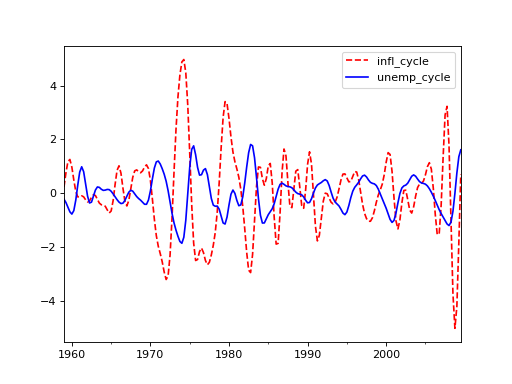# statsmodels.tsa.filters.cf_filter.cffilter¶

statsmodels.tsa.filters.cf_filter.cffilter(X, low=6, high=32, drift=True)[source]

Christiano Fitzgerald asymmetric, random walk filter

Parameters: X (array-like) – 1 or 2d array to filter. If 2d, variables are assumed to be in columns. low (float) – Minimum period of oscillations. Features below low periodicity are filtered out. Default is 6 for quarterly data, giving a 1.5 year periodicity. high (float) – Maximum period of oscillations. Features above high periodicity are filtered out. Default is 32 for quarterly data, giving an 8 year periodicity. drift (bool) – Whether or not to remove a trend from the data. The trend is estimated as np.arange(nobs)*(X[-1] - X)/(len(X)-1) cycle (array) – The features of X between periodicities given by low and high trend (array) – The trend in the data with the cycles removed.

Examples

>>> import statsmodels.api as sm
>>> import pandas as pd
>>> dta = sm.datasets.macrodata.load_pandas().data
>>> index = pd.DatetimeIndex(start='1959Q1', end='2009Q4', freq='Q')
>>> dta.set_index(index, inplace=True)

>>> cf_cycles, cf_trend = sm.tsa.filters.cffilter(dta[["infl", "unemp"]])

>>> import matplotlib.pyplot as plt
>>> fig, ax = plt.subplots()
>>> cf_cycles.plot(ax=ax, style=['r--', 'b-'])
>>> plt.show()